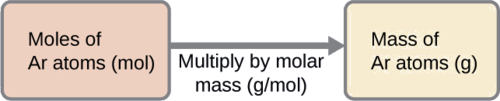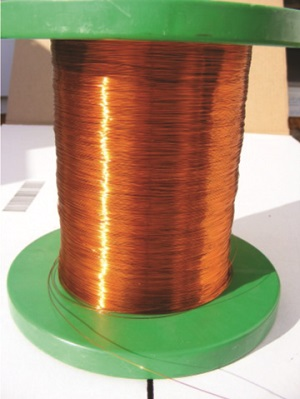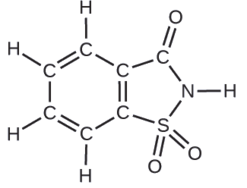# 2.4: Using the Mole in Calculations

$$\newcommand{\vecs}{\overset { \rightharpoonup} {\mathbf{#1}} }$$ $$\newcommand{\vecd}{\overset{-\!-\!\rightharpoonup}{\vphantom{a}\smash {#1}}}$$$$\newcommand{\id}{\mathrm{id}}$$ $$\newcommand{\Span}{\mathrm{span}}$$ $$\newcommand{\kernel}{\mathrm{null}\,}$$ $$\newcommand{\range}{\mathrm{range}\,}$$ $$\newcommand{\RealPart}{\mathrm{Re}}$$ $$\newcommand{\ImaginaryPart}{\mathrm{Im}}$$ $$\newcommand{\Argument}{\mathrm{Arg}}$$ $$\newcommand{\norm}{\| #1 \|}$$ $$\newcommand{\inner}{\langle #1, #2 \rangle}$$ $$\newcommand{\Span}{\mathrm{span}}$$ $$\newcommand{\id}{\mathrm{id}}$$ $$\newcommand{\Span}{\mathrm{span}}$$ $$\newcommand{\kernel}{\mathrm{null}\,}$$ $$\newcommand{\range}{\mathrm{range}\,}$$ $$\newcommand{\RealPart}{\mathrm{Re}}$$ $$\newcommand{\ImaginaryPart}{\mathrm{Im}}$$ $$\newcommand{\Argument}{\mathrm{Arg}}$$ $$\newcommand{\norm}{\| #1 \|}$$ $$\newcommand{\inner}{\langle #1, #2 \rangle}$$ $$\newcommand{\Span}{\mathrm{span}}$$$$\newcommand{\AA}{\unicode[.8,0]{x212B}}$$

## The Mole

The identity of a substance is defined not only by the types of atoms or ions it contains, but by the quantity of each type of atom or ion. For example, water, H2O, and hydrogen peroxide, H2O2, are alike in that their respective molecules are composed of hydrogen and oxygen atoms. However, because a hydrogen peroxide molecule contains two oxygen atoms, as opposed to the water molecule, which has only one, the two substances exhibit very different properties. Today, we possess sophisticated instruments that allow the direct measurement of these defining microscopic traits; however, the same traits were originally derived from the measurement of macroscopic properties (the masses and volumes of bulk quantities of matter) using relatively simple tools (balances and volumetric glassware). This experimental approach required the introduction of a new unit for amount of substances, the mole, which remains indispensable in modern chemical science.

The mole is an amount unit similar to familiar units like pair, dozen, gross, etc. It provides a specific measure of the number of atoms or molecules in a bulk sample of matter. A mole is defined as the amount of substance containing the same number of discrete entities (such as atoms, molecules, and ions) as the number of atoms in a sample of pure 12C weighing exactly 12 g. One Latin connotation for the word “mole” is “large mass” or “bulk,” which is consistent with its use as the name for this unit. The mole provides a link between an easily measured macroscopic property, bulk mass, and an extremely important fundamental property, number of atoms, molecules, and so forth.

The number of entities composing a mole has been experimentally determined to be $$6.02214179 \times 10^{23}$$, a fundamental constant named Avogadro’s number (NA) or the Avogadro constant in honor of Italian scientist Amedeo Avogadro. This constant is properly reported with an explicit unit of “per mole,” a conveniently rounded version being 6.022 \times 10^{23}\/ce{mol}\).

Video $$\PageIndex{1}$$: What is Avogadro's Number?

Consistent with its definition as an amount unit, 1 mole of any element contains the same number of atoms as 1 mole of any other element. The masses of 1 mole of different elements, however, are different, since the masses of the individual atoms are drastically different. The molar mass of an element (or compound) is the mass in grams of 1 mole of that substance, a property expressed in units of grams per mole (g/mol) (Figure $$\PageIndex{1}$$).Figure $$\PageIndex{1}$$: Each sample contains $$6.022 \times 10^{23}$$ atoms —1.00 mol of atoms. From left to right (top row): 65.4 g zinc, 12.0 g carbon, 24.3 g magnesium, and 63.5 g copper. From left to right (bottom row): 32.1 g sulfur, 28.1 g silicon, 207 g lead, and 118.7 g tin. (credit: modification of work by Mark Ott).

Because the definitions of both the mole and the atomic mass unit are based on the same reference substance, 12C, the molar mass of any substance is numerically equivalent to its atomic or formula weight in amu. Per the amu definition, a single 12C atom weighs 12 amu (its atomic mass is 12 amu). The former definition of the mole was that a mole was 12 g of 12C contains 1 mole of 12C atoms (its molar mass is 12 g/mol). This relationship holds for all elements, since their atomic masses are measured relative to that of the amu-reference substance, 12C. Extending this principle, the molar mass of a compound in grams is likewise numerically equivalent to its formula mass in amu. On May 20, 2019 the definition was permanently changed to Avogadro's number: a mole is $$6.02214179 \times 10^{23}$$ of any object, from atoms to apples.1

Table $$\PageIndex{2}$$: Mass of one mole of elements
Element Average Atomic Mass (amu) Molar Mass (g/mol) Atoms/Mole
C 12.01 12.01 $$6.022 \times 10^{23}$$
H 1.008 1.008 $$6.022 \times 10^{23}$$
O 16.00 16.00 $$6.022 \times 10^{23}$$
Na 22.99 22.99 $$6.022 \times 10^{23}$$
Cl 33.45 35.45 $$6.022 \times 10^{23}$$

While atomic mass and molar mass are numerically equivalent, keep in mind that they are vastly different in terms of scale, as represented by the vast difference in the magnitudes of their respective units (amu versus g). To appreciate the enormity of the mole, consider a small drop of water after a rainfall. Although this represents just a tiny fraction of 1 mole of water (~18 g), it contains more water molecules than can be clearly imagined. If the molecules were distributed equally among the roughly seven billion people on earth, each person would receive more than 100 billion molecules.

Video $$\PageIndex{2}$$: The mole is used in chemistry to represent $$6.022 \times 10^{23}$$ of something, but it can be difficult to conceptualize such a large number. Watch this video and then complete the “Think” questions that follow. Explore more about the mole by reviewing the information under “Dig Deeper.”

The relationships between formula mass, the mole, and Avogadro’s number can be applied to compute various quantities that describe the composition of substances and compounds. For example, if we know the mass and chemical composition of a substance, we can determine the number of moles and calculate number of atoms or molecules in the sample. Likewise, if we know the number of moles of a substance, we can derive the number of atoms or molecules and calculate the substance’s mass.

Example $$\PageIndex{1}$$: Deriving Moles from Grams for an Element

According to nutritional guidelines from the US Department of Agriculture, the estimated average requirement for dietary potassium is 4.7 g. What is the estimated average requirement of potassium in moles?

Solution

The mass of K is provided, and the corresponding amount of K in moles is requested. Referring to the periodic table, the atomic mass of K is 39.10 amu, and so its molar mass is 39.10 g/mol. The given mass of K (4.7 g) is a bit more than one-tenth the molar mass (39.10 g), so a reasonable “ballpark” estimate of the number of moles would be slightly greater than 0.1 mol.

The molar amount of a substance may be calculated by dividing its mass (g) by its molar mass (g/mol):The factor-label method supports this mathematical approach since the unit “g” cancels and the answer has units of “mol:”

$\mathrm{4.7\; \cancel{g} K \left ( \dfrac{mol\; K}{39.10\;\cancel{g}}\right)=0.12\;mol\; K} \nonumber$

The calculated magnitude (0.12 mol K) is consistent with our ballpark expectation, since it is a bit greater than 0.1 mol.

Exercise $$\PageIndex{1}$$: Beryllium

Beryllium is a light metal used to fabricate transparent X-ray windows for medical imaging instruments. How many moles of Be are in a thin-foil window weighing 3.24 g?

0.360 mol

Example $$\PageIndex{2}$$: Deriving Grams from Moles for an Element

A liter of air contains $$9.2 \times 10^{−4}$$ mol argon. What is the mass of Ar in a liter of air?

Solution

The molar amount of Ar is provided and must be used to derive the corresponding mass in grams. Since the amount of Ar is less than 1 mole, the mass will be less than the mass of 1 mole of Ar, approximately 40 g. The molar amount in question is approximately one-one thousandth (~10−3) of a mole, and so the corresponding mass should be roughly one-one thousandth of the molar mass (~0.04 g):In this case, logic dictates (and the factor-label method supports) multiplying the provided amount (mol) by the molar mass (g/mol):

$\mathrm{9.2 \times10^{-4}\; \cancel{mol} \; Ar \left( \dfrac{39.95\;g}{\cancel{mol}\;Ar} \right)=0.037\;g\; Ar} \nonumber$

The result is in agreement with our expectations, around 0.04 g Ar.

Exercise $$\PageIndex{2}$$

What is the mass of 2.561 mol of gold?

504.4 g

Example $$\PageIndex{3}$$: Deriving Number of Atoms from Mass for an Element

Copper is commonly used to fabricate electrical wire (Figure $$\PageIndex{6}$$). How many copper atoms are in 5.00 g of copper wire?Figure $$\PageIndex{6}$$: Copper wire is composed of many, many atoms of Cu. (credit: Emilian Robert Vicol)

Solution

The number of Cu atoms in the wire may be conveniently derived from its mass by a two-step computation: first calculating the molar amount of Cu, and then using Avogadro’s number (NA) to convert this molar amount to number of Cu atoms:Considering that the provided sample mass (5.00 g) is a little less than one-tenth the mass of 1 mole of Cu (~64 g), a reasonable estimate for the number of atoms in the sample would be on the order of one-tenth NA, or approximately 1022 Cu atoms. Carrying out the two-step computation yields:

$\mathrm{5.00\:\cancel{g}\:Cu\left(\dfrac{\cancel{mol}\:Cu}{63.55\:\cancel{g}}\right)\left(\dfrac{6.022\times10^{23}\:atoms}{\cancel{mol}}\right)=4.74\times10^{22}\:atoms\: of\: copper}$

The factor-label method yields the desired cancellation of units, and the computed result is on the order of 1022 as expected.

Exercise $$\PageIndex{3}$$

A prospector panning for gold in a river collects 15.00 g of pure gold. How many Au atoms are in this quantity of gold?

$$4.586 \times 10^{22}\; Au$$ atoms

Example $$\PageIndex{4}$$: Deriving Moles from Grams for a Compound

Our bodies synthesize protein from amino acids. One of these amino acids is glycine, which has the molecular formula C2H5O2N. How many moles of glycine molecules are contained in 28.35 g of glycine?

Solution

We can derive the number of moles of a compound from its mass following the same procedure we used for an element in Example $$\PageIndex{6}$$:The molar mass of glycine is required for this calculation, and it is computed in the same fashion as its molecular mass. One mole of glycine, C2H5O2N, contains 2 moles of carbon, 5 moles of hydrogen, 2 moles of oxygen, and 1 mole of nitrogen:The provided mass of glycine (~28 g) is a bit more than one-third the molar mass (~75 g/mol), so we would expect the computed result to be a bit greater than one-third of a mole (~0.33 mol). Dividing the compound’s mass by its molar mass yields:

$\mathrm{28.35\:\cancel{g}\:glycine\left(\dfrac{mol\: glycine}{75.07\:\cancel{g}}\right)=0.378\:mol\: glycine} \nonumber$

This result is consistent with our rough estimate.

Exercise $$\PageIndex{4}$$

How many moles of sucrose, $$C_{12}H_{22}O_{11}$$, are in a 25-g sample of sucrose?

0.073 mol

Example $$\PageIndex{5}$$: Deriving Grams from Moles for a Compound

Vitamin C is a covalent compound with the molecular formula C6H8O6. The recommended daily dietary allowance of vitamin C for children aged 4–8 years is 1.42 × 10−4 mol. What is the mass of this allowance in grams?

Solution

As for elements, the mass of a compound can be derived from its molar amount as shown:The molar mass for this compound is computed to be 176.124 g/mol. The given number of moles is a very small fraction of a mole (~10−4 or one-ten thousandth); therefore, we would expect the corresponding mass to be about one-ten thousandth of the molar mass (~0.02 g). Performing the calculation, we get:

$\mathrm{1.42\times10^{-4}\:\cancel{mol}\:vitamin\: C\left(\dfrac{176.124\:g}{\cancel{mol}\:vitamin\: C}\right)=0.0250\:g\: vitamin\: C} \nonumber$

This is consistent with the anticipated result.

Exercise $$\PageIndex{5}$$

What is the mass of 0.443 mol of hydrazine, $$N_2H_4$$?

14.2 g

Example $$\PageIndex{6}$$: Deriving the Number of Molecules from the Compound Mass

A packet of an artificial sweetener contains 40.0 mg of saccharin (C7H5NO3S), which has the structural formula:Given that saccharin has a molar mass of 183.18 g/mol, how many saccharin molecules are in a 40.0-mg (0.0400-g) sample of saccharin? How many carbon atoms are in the same sample?

Solution

The number of molecules in a given mass of compound is computed by first deriving the number of moles, as demonstrated in Example $$\PageIndex{8}$$, and then multiplying by Avogadro’s number:Using the provided mass and molar mass for saccharin yields:

$\mathrm{0.0400\:\cancel{g}\:\ce{C7H5NO3S}\left(\dfrac{\cancel{mol}\:\ce{C7H5NO3S}}{183.18\:\cancel{g}\:\ce{C7H5NO3S}}\right)\left(\dfrac{6.022\times10^{23}\:\ce{C7H5NO3S}\:molecules}{1\:\cancel{mol}\:\ce{C7H5NO3S}}\right)}\\ =\mathrm{1.31\times10^{20}\:\ce{C7H5NO3S}\:molecules}$

The compound’s formula shows that each molecule contains seven carbon atoms, and so the number of C atoms in the provided sample is:

$\mathrm{1.31\times10^{20}\:\ce{C7H5NO3S}\: molecules\left(\dfrac{7\:C\: atoms}{1\:\ce{C7H5NO3S}\: molecule}\right)=9.20\times10^{21}\:C\: atoms} \nonumber$

Exercise $$\PageIndex{6}$$

How many $$C_4H_{10}$$ molecules are contained in 9.213 g of this compound? How many hydrogen atoms?

• $$9.545 \times 10^{22}\; \text{molecules}\; C_4H_{10}$$
• $$9.545 \times 10^{23 }\;\text{atoms}\; H$$

## Summary

Video $$\PageIndex{5}$$: A preview of some of the uses we will have for moles in upcoming units

## Glossary

mole
amount of substance containing the same number of atoms, molecules, ions, or other entities as the number of atoms in exactly 12 grams of 12C